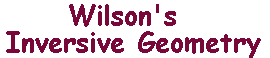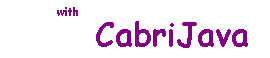The angle between two arcs

 Suppose that we want to define the signed angle between arcs PA and PB of curves meeting at a point P. Provided the curves have tangents at P, the sensible way to define the angle is as the angle between the corresponding tangent rays. Take a point Q on PA. Provided Q is close to P, the circle, centre P, radius PQ should cross the arc PB at some point, R say. The rays PQ and PR define the angle QPR at P. As Q approaches P along the arc PA, R will approach P along arc PB. In the limit, PQ approaches the tangent ray to arc PA at P, and PR the tangent ray to PB at P. In the picture, the curves and corresponding tangent rays are red and green. To see this in the CabriJava box, drag the point Q towards P (it will stay on the arc PA). You should see the rays PQ and PR approach the tangents.

The Invariance Theorem

 Let C be a circle with centre O. Suppose that two arcs meet in a point P, so they define an angle at P. We consider inversion with respect to C. The inverses of the arcs will be arcs meeting at P' (the inverse of P). It is a remarkable fact that the angle between the inverse arcs is always the reverse of the angle between the original arcs. The Invariance Theorem Inversion in a circle preserves the size of any angle, but reverses the sense. The CabriJava box on the right illustrates the Theorem, and suggests the proof! If you drag Q towards P (it stays on the upper green arc), Then R, the point where OQ meets the upper red arc, also moves towards P. The inverses are respectively Q' and R', and these move along the inverse arcs towards P'. Of course, the rays PQ, PR, P'Q' and P'R' tend towards the tangents at the respective points.